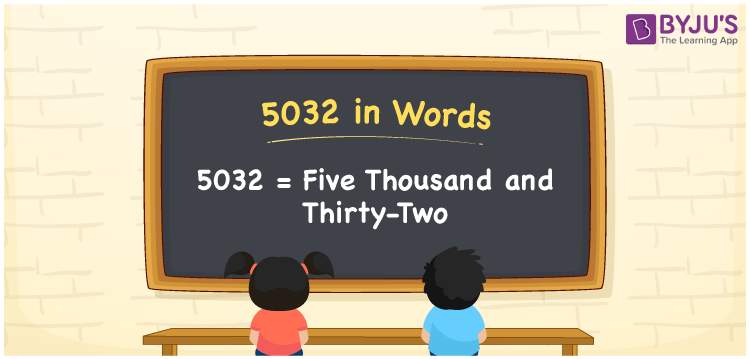# 5032 in Words

The number 5032 in words is written as Five thousand thirty-two. In both the International and Indian System of Numerals, 5032 is written as 5,032 and read as Five thousand thirty-two. We may use 5032 as “that gadget costs 5032 rupees” in this context, 5032 represents an amount; that is why 5032 is a Cardinal Number since it describes some quantity.

 5032 in Words Five thousand thirty-two Five thousand thirty-two in Numbers 5032

## 5032 in English Words

In English words, 5032 is written as “Five thousand thirty-two”.How to Write 5032 in Words?

To write 5032 in words, we shall use the place value. In the place value chart, write 5 in thousands, 0 in hundreds, 3 in tens and 2 in ones. Let us make a place value chart to write the number 5032 in words.

 Thousands Hundreds Tens Ones 5 0 3 2

Thus, we can the expanded form as

5 × Thousand + 0 × Hundred + 3 × Ten + 2 × One

= 5 × 1000 + 0 × 100 + 3 × 10 + 2 × 1

= 5000 + 0 + 30 + 2

= 5032

= Five thousand thirty-two.

5032 is a Natural Number, the successor of 5031 and the predecessor of 5033

5032 in words – Five thousand thirty-two

• Is 5032 an odd number? – No
• Is 5032 an even number? – Yes
• Is 5032 a perfect square number? – No
• Is 5032 a perfect cube number? – No
• Is 5032 a prime number? – No
• Is 5032 a composite number? – Yes

## Frequently Asked Questions on 5032 in Words

Q1

### How to write 5032 in words?

5032 in words is written as Five thousand thirty-two.
Q2

### How to write 5032 in the International and Indian System of Numerals?

In both, the system of numerals, 5032 in words, is written as Five thousand thirty-two.
Q3

### How to write 5032 in a place value chart?

In the place value chart, write 5 in thousands, 0 in hundreds, 3 in tens and 2 in ones, respectively.